#Function Repository Resource:

# CurvaturePlot

Plot a curve defined by its curvature

Contributed by: Sander Huisman
 ResourceFunction["CurvaturePlot"][f,{t,tmin,tmax}] plots the curve defined by its curvature f as a function of t. ResourceFunction["CurvaturePlot"][{f1,f2,…},{t,tmin,tmax}] plots several curves defined by their curvatures fi. ResourceFunction["CurvaturePlot"][…,{t,tmin,tmax},{{x0,y0},θ0}] starts the curves at {x0,y0} in the direction θ0.

## Details and Options

The default starting location is at {0,0} and in the right direction (θ0=0). The angle θ0 is defined as follows: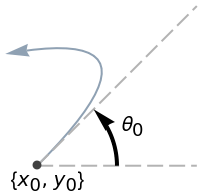A constant curvature c draws a circle with radius 1/c.
ResourceFunction["CurvaturePlot"] has the same options as ParametricPlot and NDSolve.

## Examples

### Basic Examples

Plot a curve with increasing curvature:

 In:=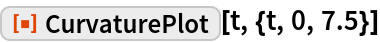Out=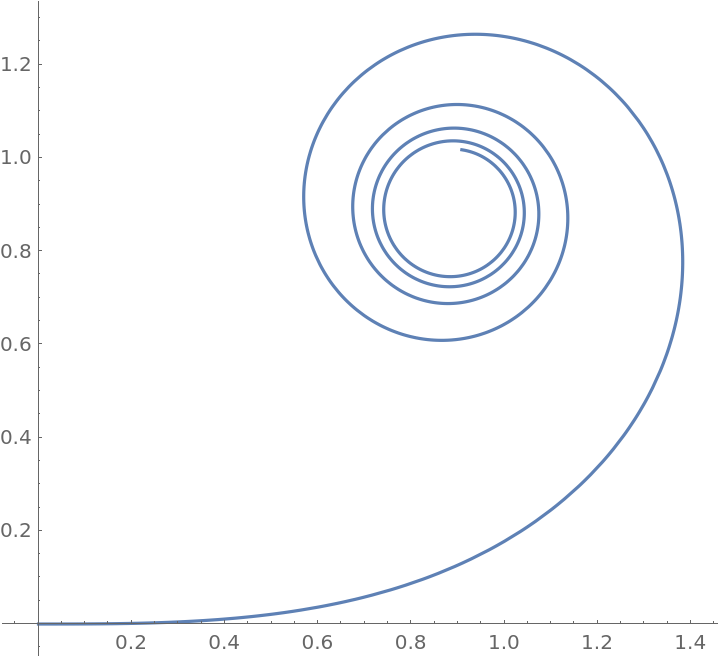Plot a curve with a sinusoidally varying curvature:

 In:=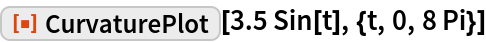Out=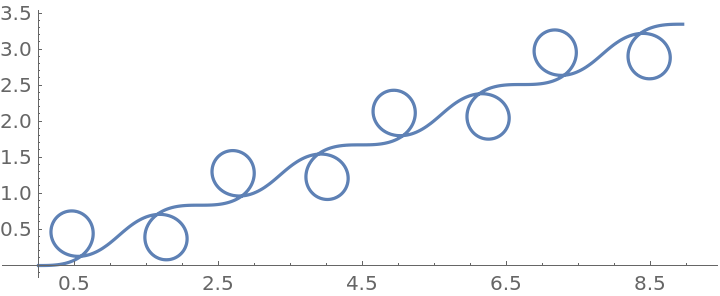Plot multiple curves:

 In:=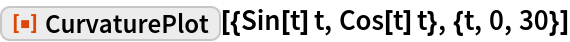Out=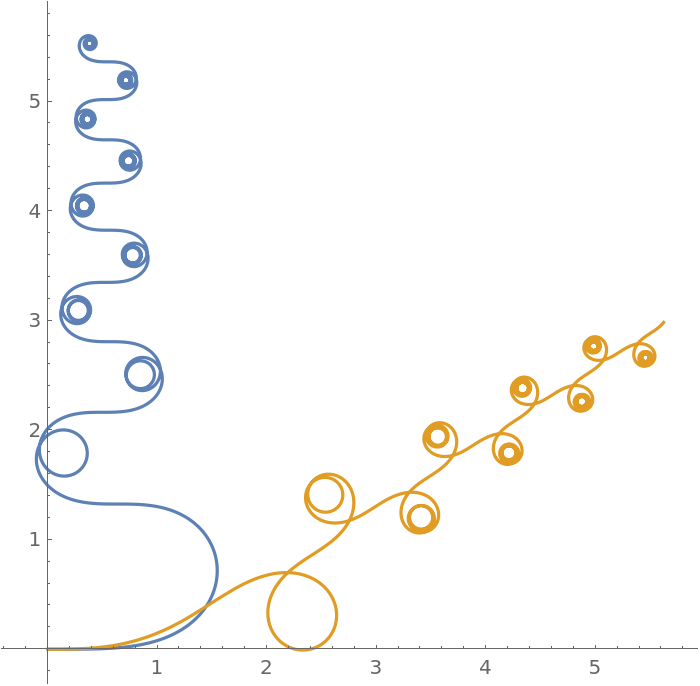### Scope

Start at the point {5,7} in the left direction:

 In:=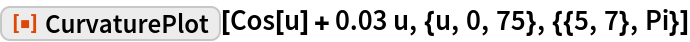Out=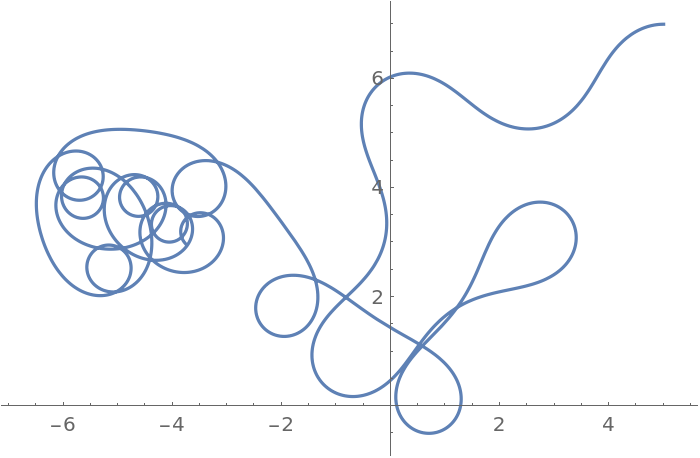### Options

#### AspectRatio

By default, AspectRatio comes from PlotRange:

 In:=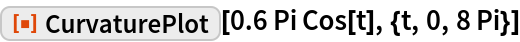Out=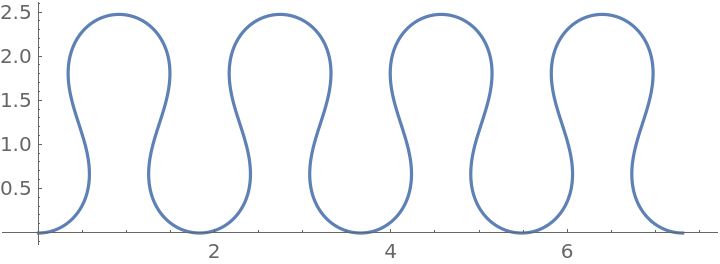Set a different AspectRatio:

 In:=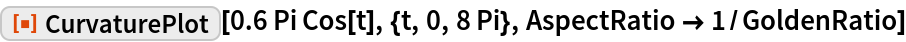Out=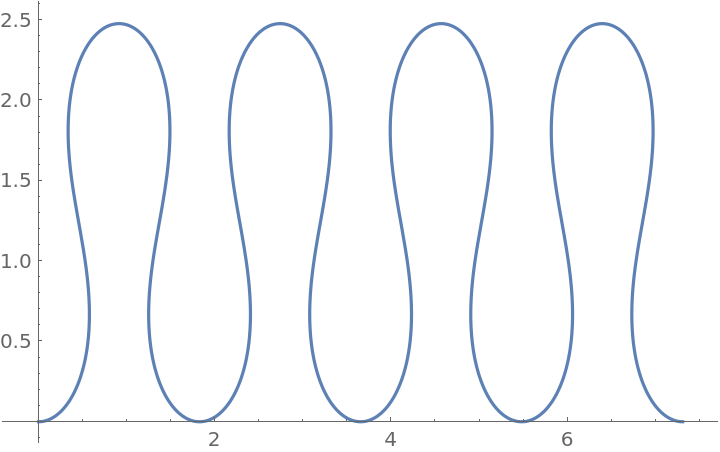#### Axes

Draw no axes:

 In:=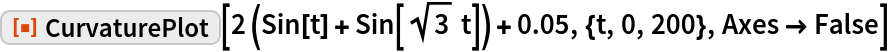Out=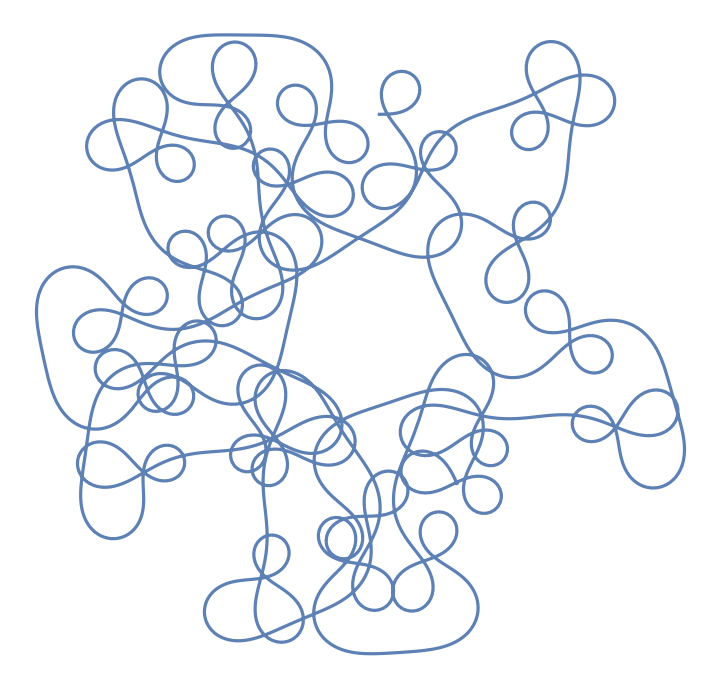#### AxesLabel

Specify labels for the x and y axes:

 In:=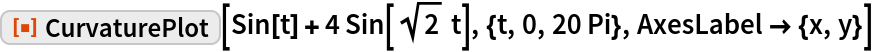Out=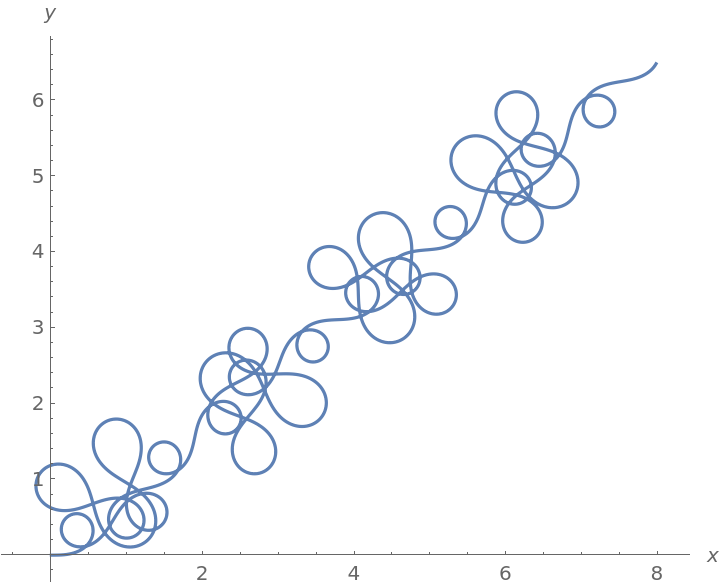#### AxesOrigin

Determine where the axes cross automatically:

 In:=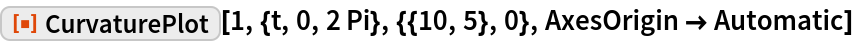Out=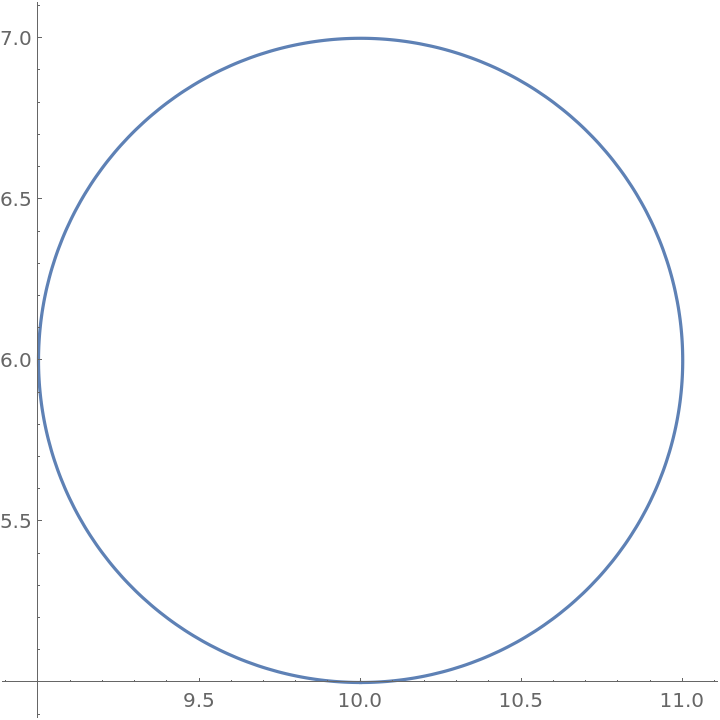Specify the axes origin at the point {0,0}:

 In:=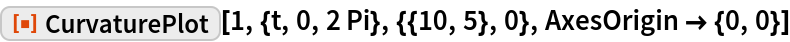Out=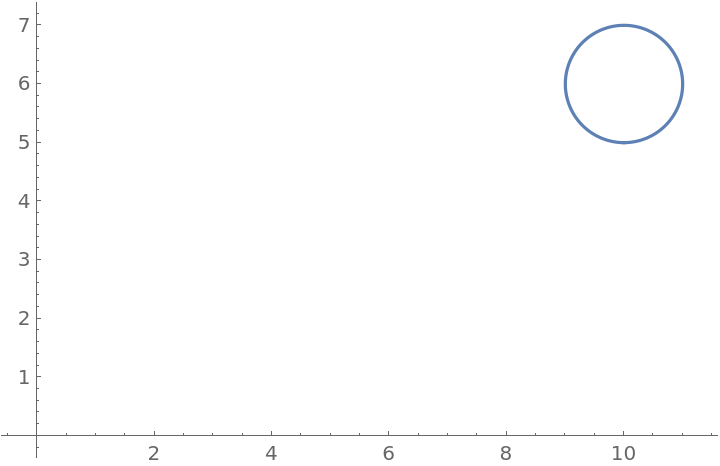#### ColorFunction

Color the curve by scaled x, y or t values:

 In:=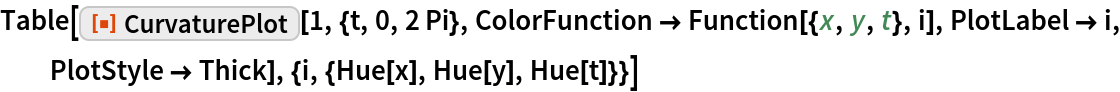Out=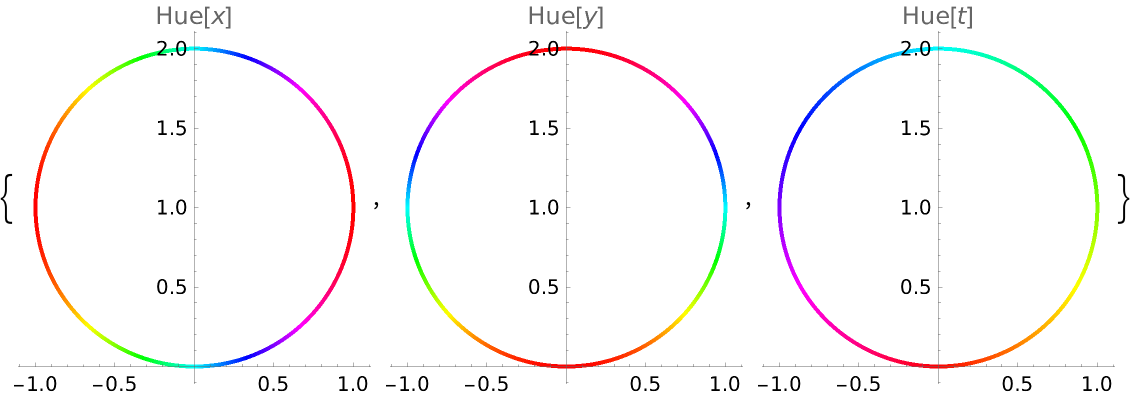In:=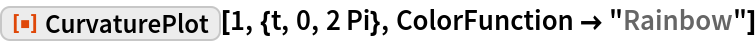Out=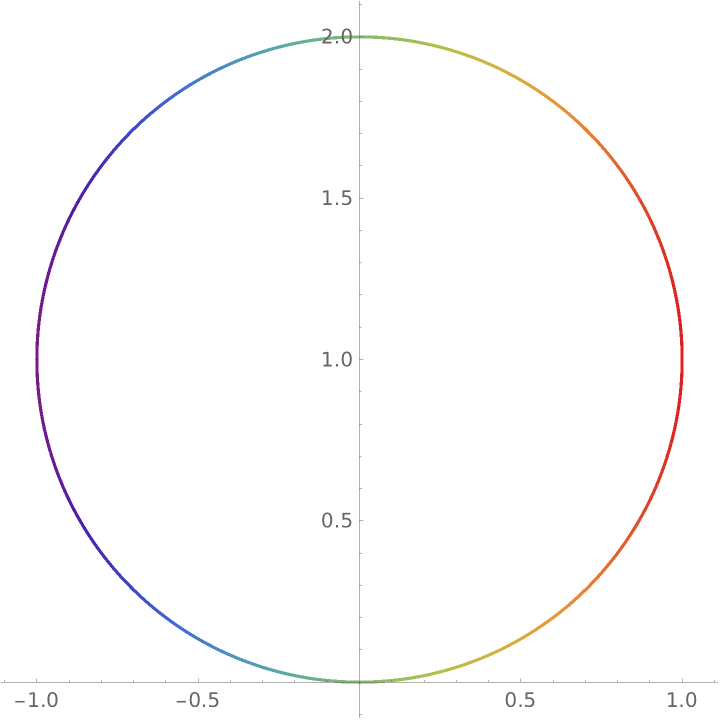ColorFunction has higher priority than PlotStyle:

 In:=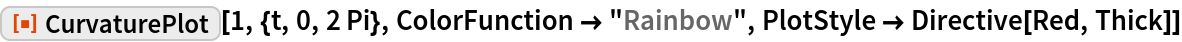Out=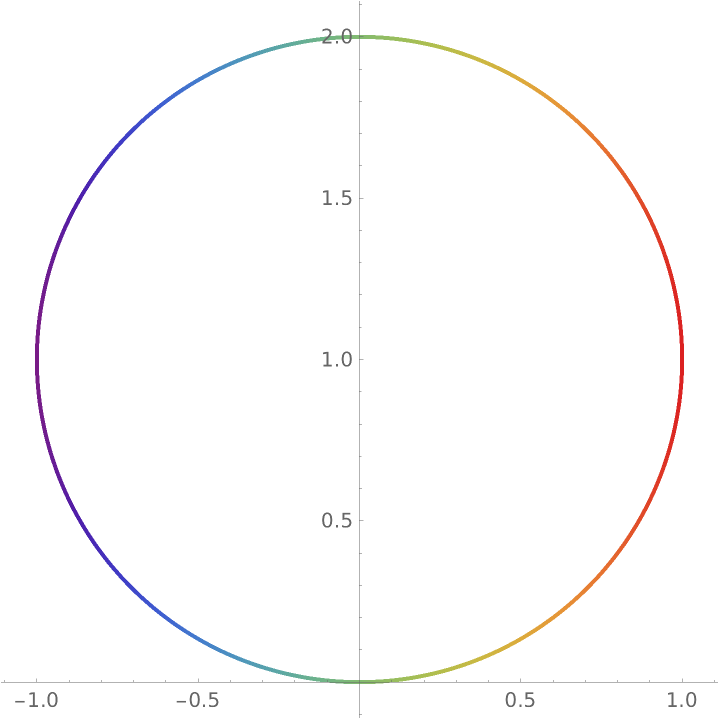Use red for the parameter t>2π:

 In:=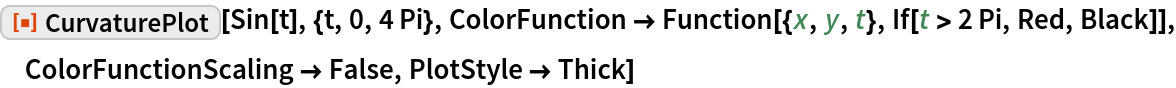Out=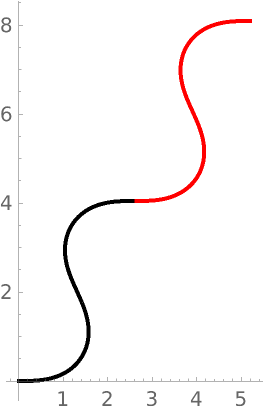Color by the absolute curvature:

 In:=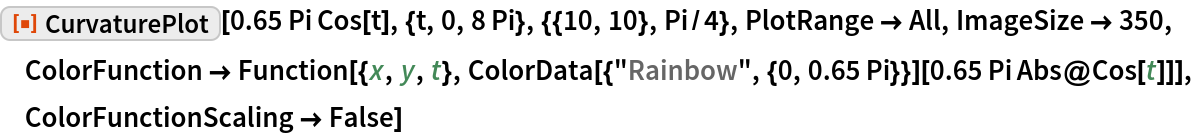Out=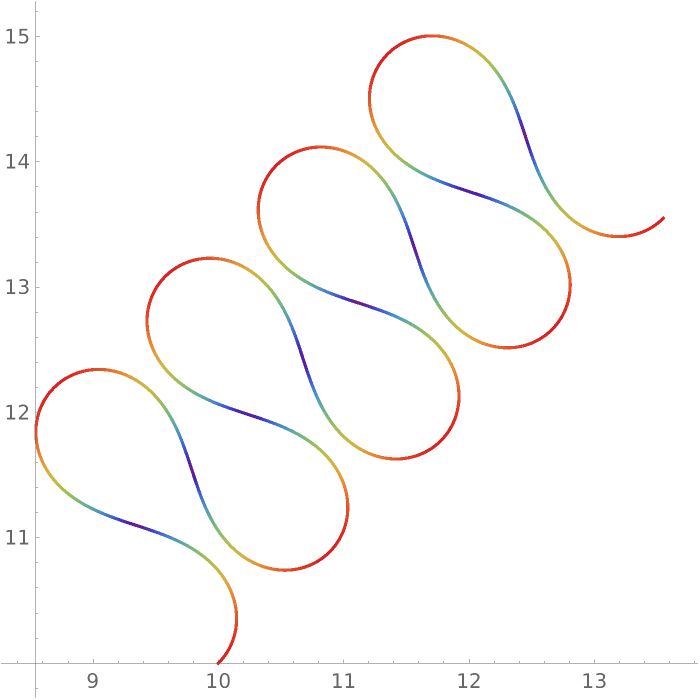#### ColorFunctionScaling

Color the curve by the phase of the sine:

 In:=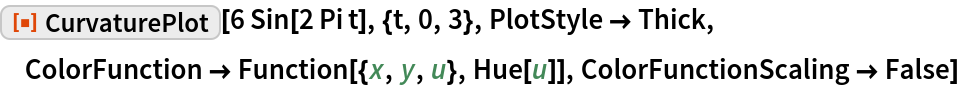Out=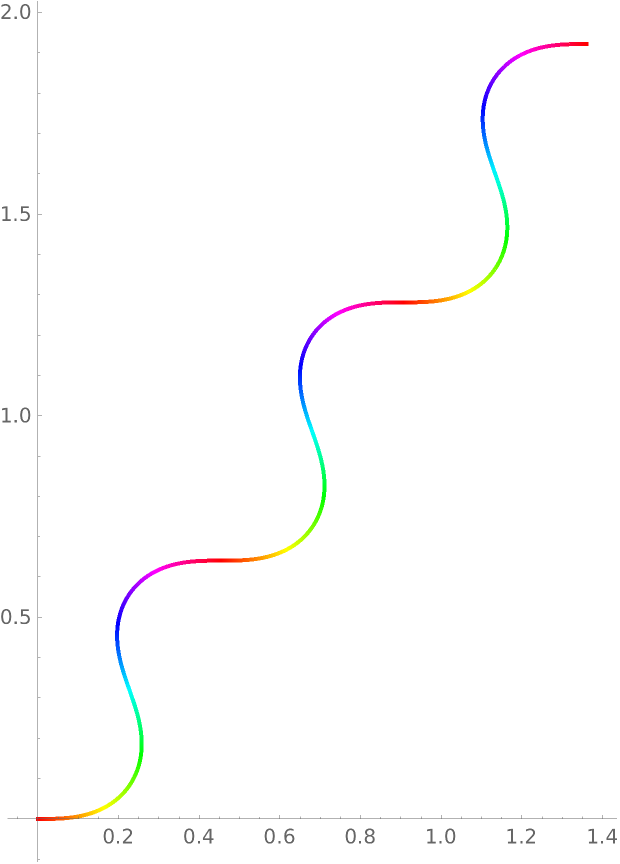#### MaxRecursion

Each level of MaxRecursion will adaptively subdivide the initial mesh into a finer mesh:

 In:=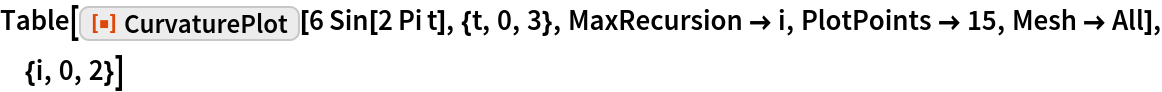Out=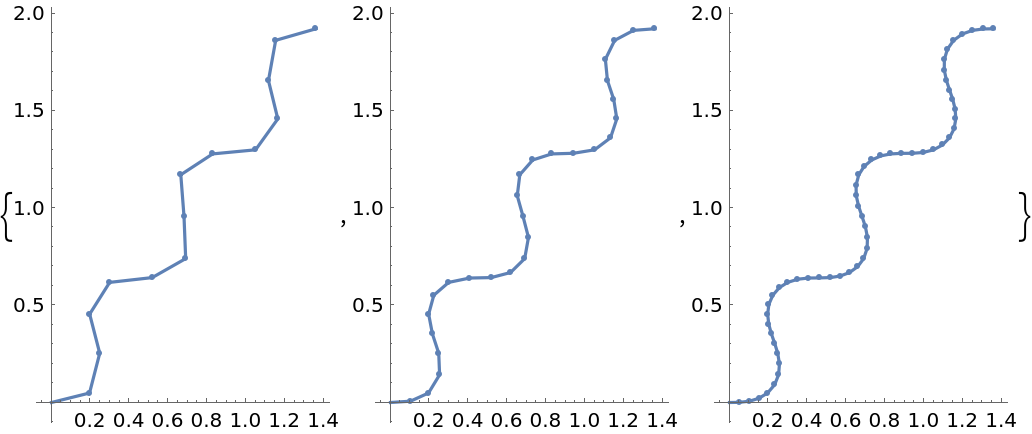#### Mesh

Show the initial and final sampling meshes:

 In:=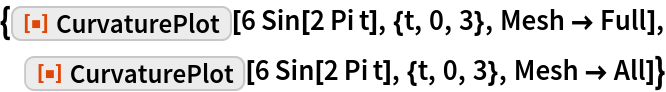Out=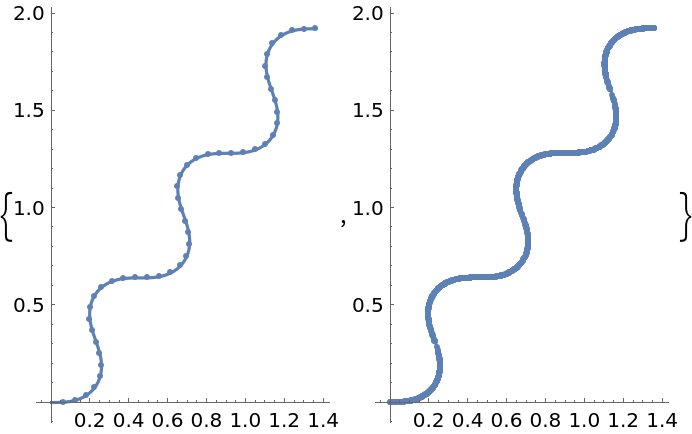#### PerformanceGoal

Generate a higher-quality plot:

 In:=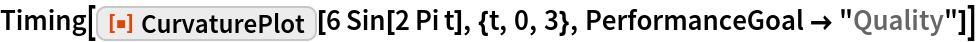Out=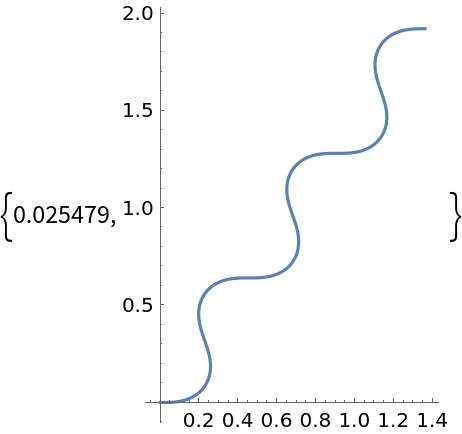Emphasize performance, possibly at the cost of quality:

 In:=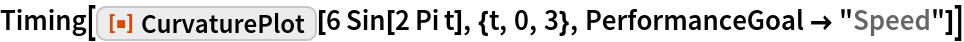Out=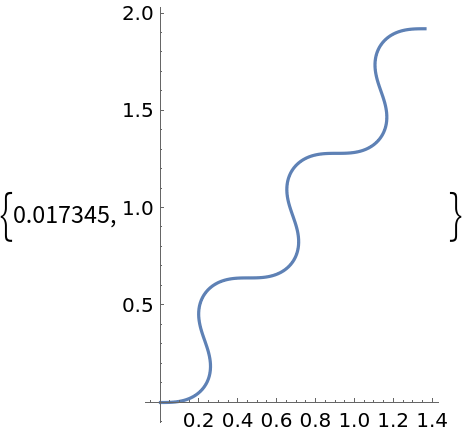#### PlotLabels

Specify the text to label the curves:

 In:=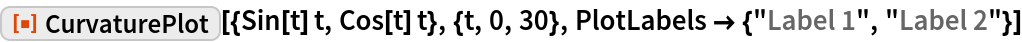Out=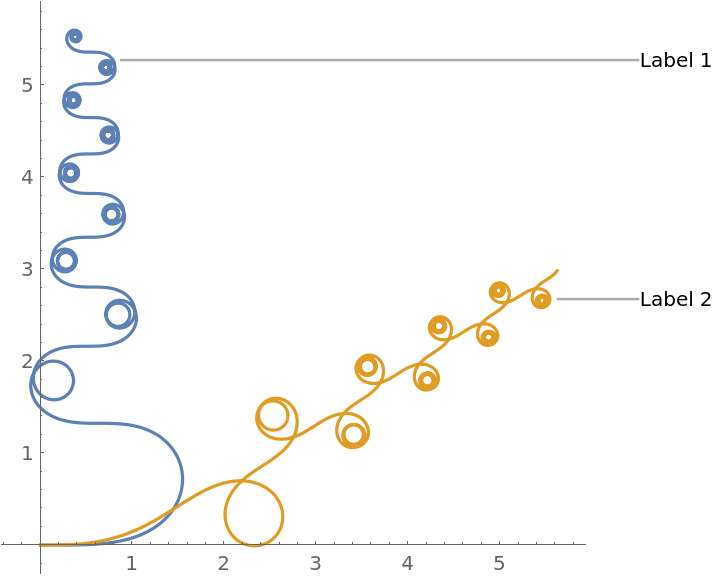Place the labels above the curves:

 In:=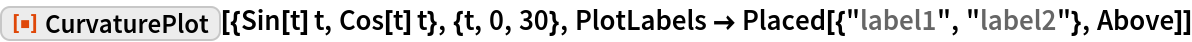Out=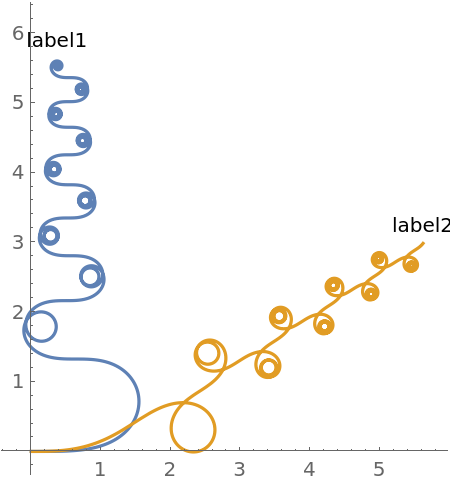Place the labels differently for each curve:

 In:=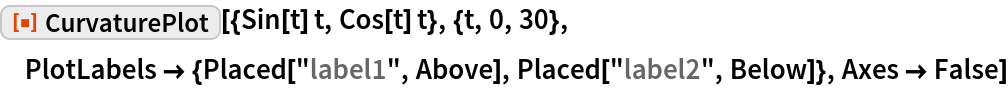Out=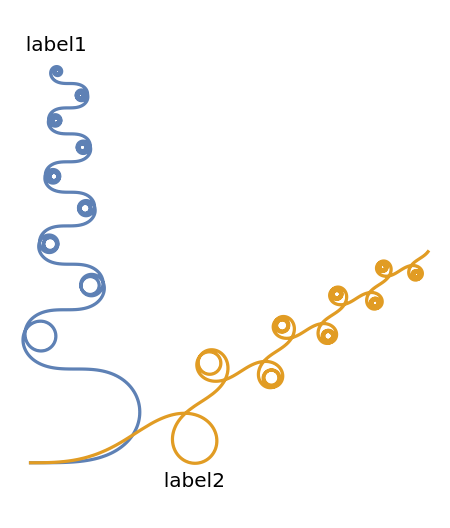Use callouts to identify the curves:

 In:=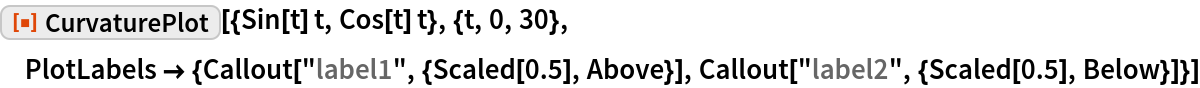Out=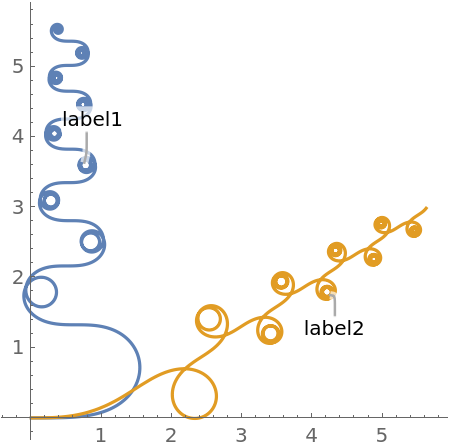Put labels relative to the outside of the curves:

 In:=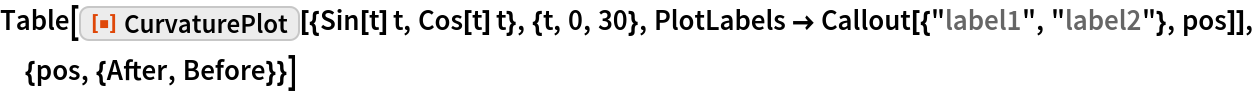Out=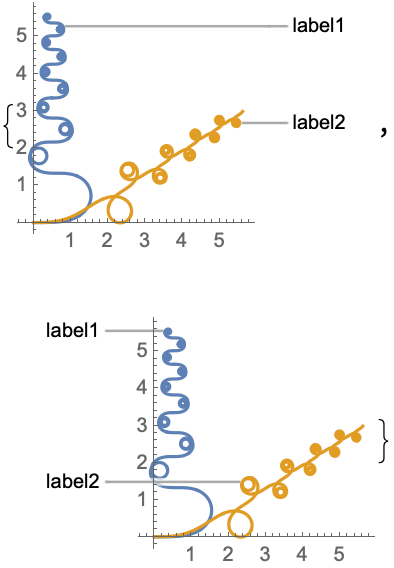Use None to not add a label:

 In:=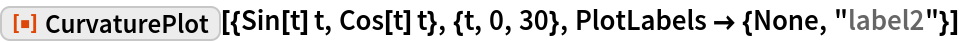Out=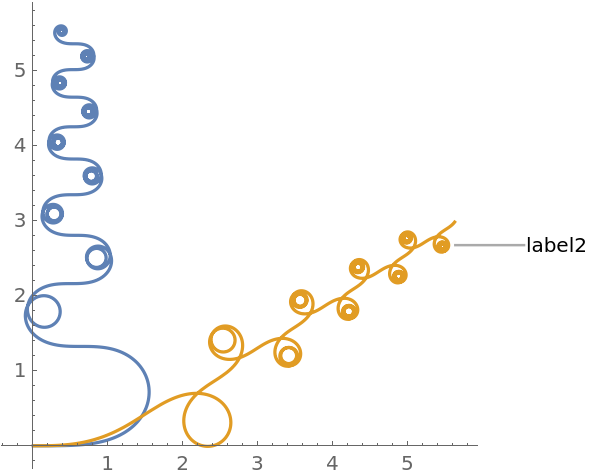#### PlotLegends

No legends are used by default:

 In:=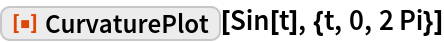Out=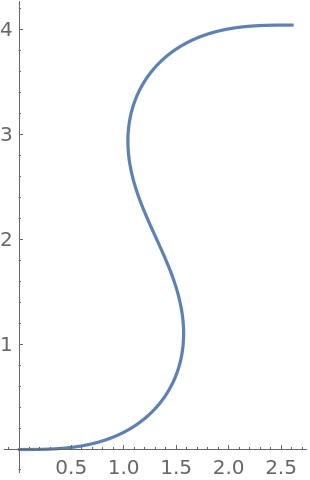Create a legend with specific labels:

 In:=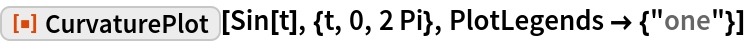Out=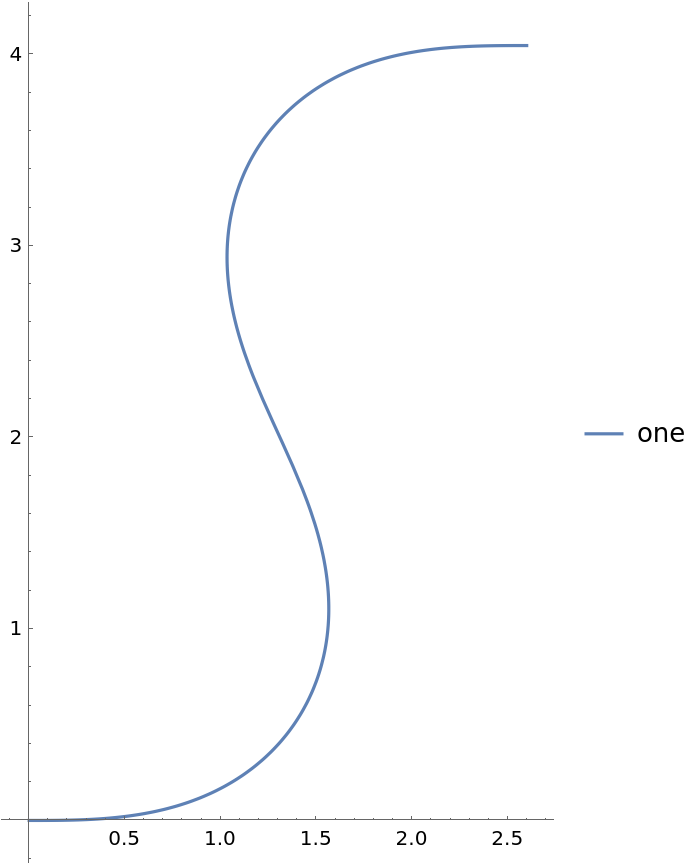PlotLegends picks up PlotStyle values automatically:

 In:=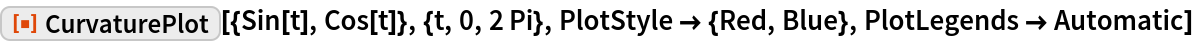Out=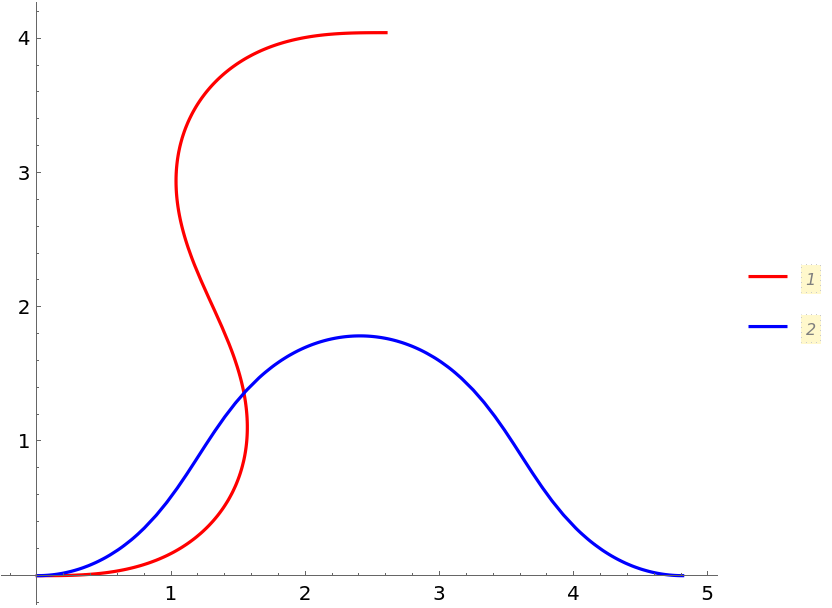Use Placed to position legends:

 In:=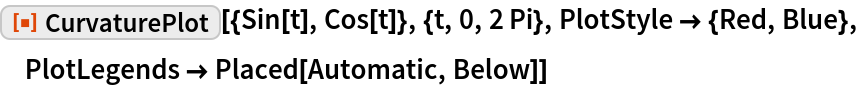Out=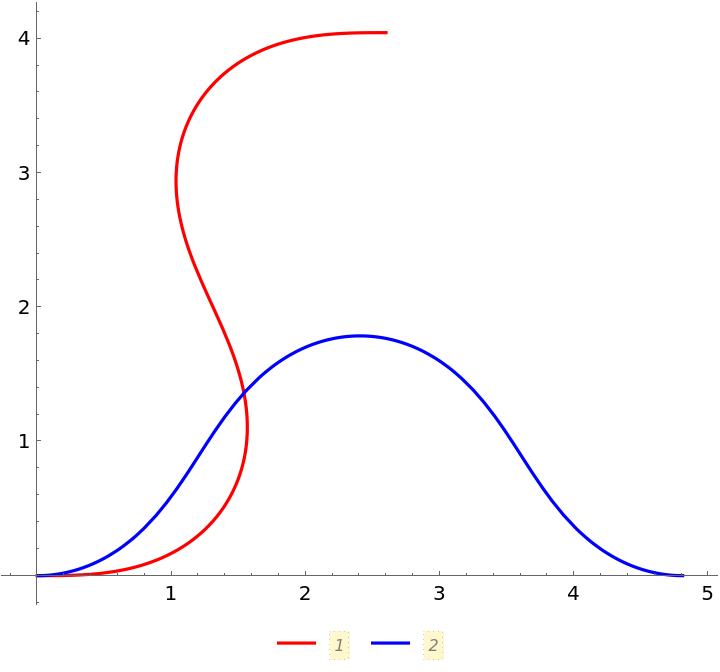Place legends inside:

 In:=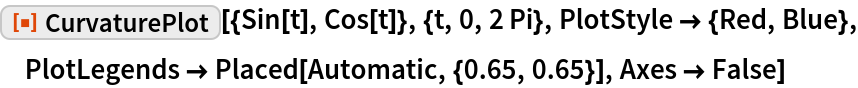Out=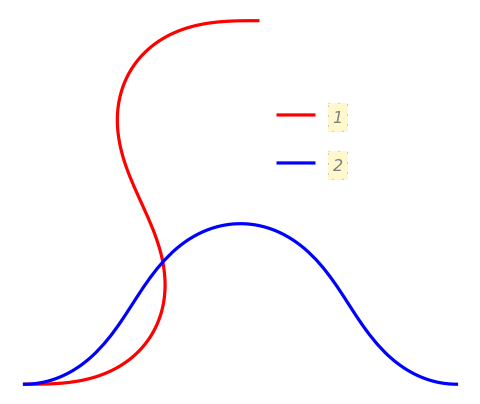Use LineLegend to modify the appearance of the legend:

 In:=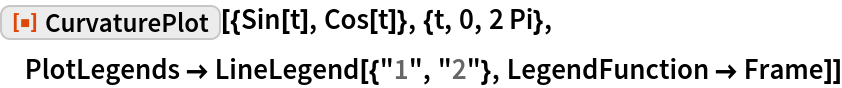Out=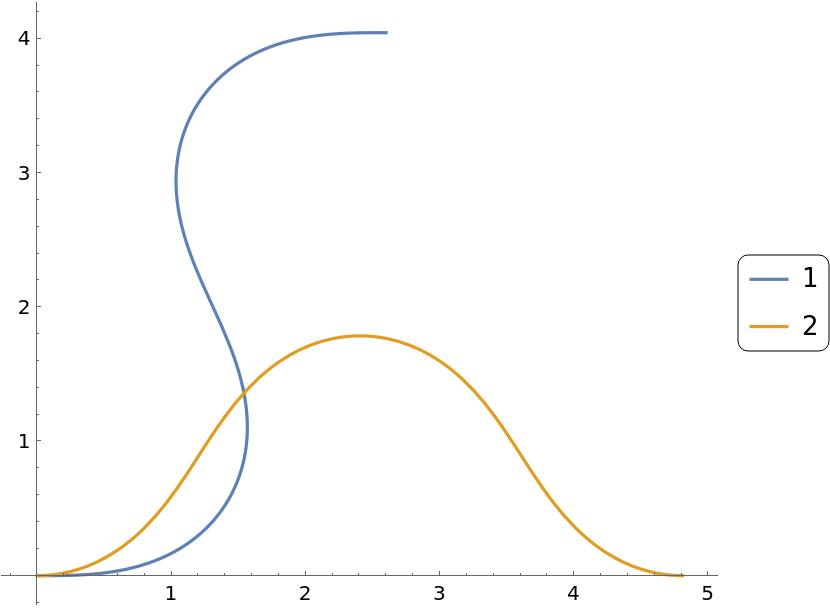#### PlotPoints

Use more initial points to get a smoother plot:

 In:=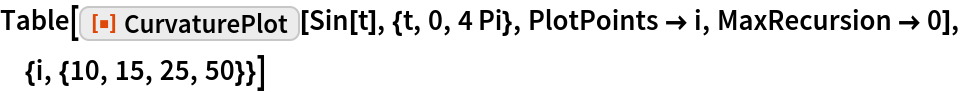Out=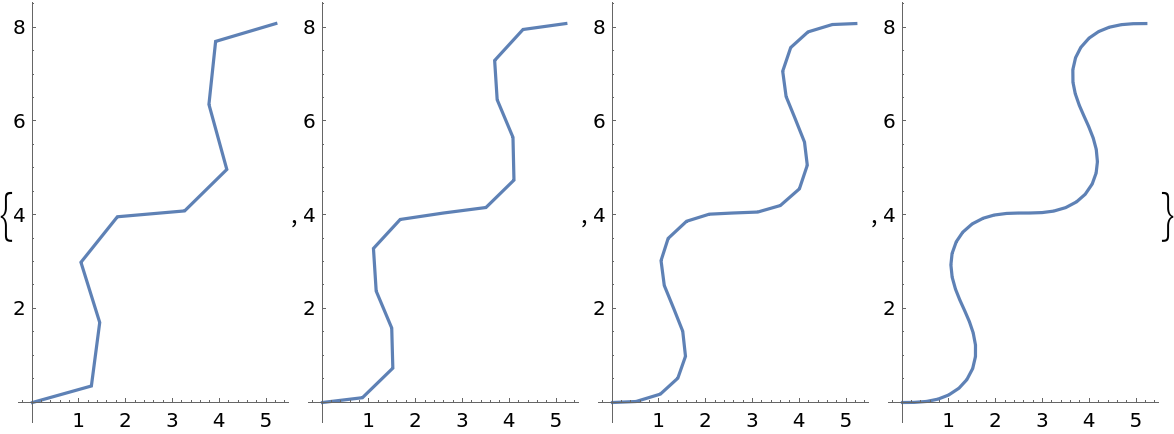#### PlotRange

Change the PlotRange:

 In:=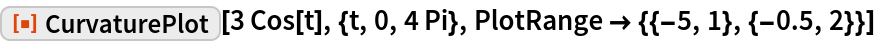Out=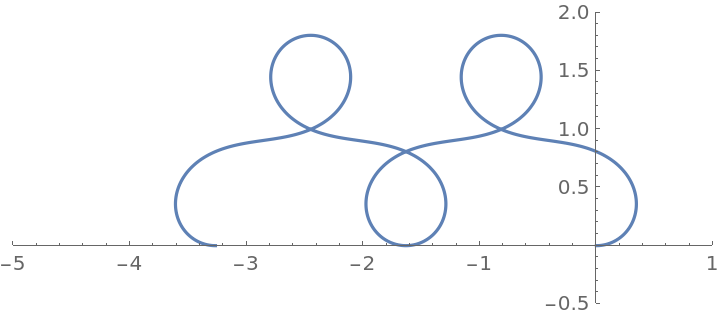#### PlotStyle

Use different style directives:

 In:=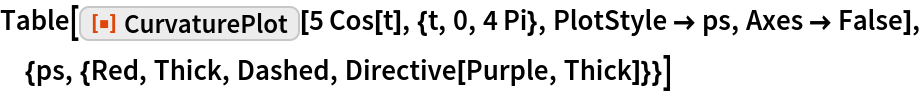Out=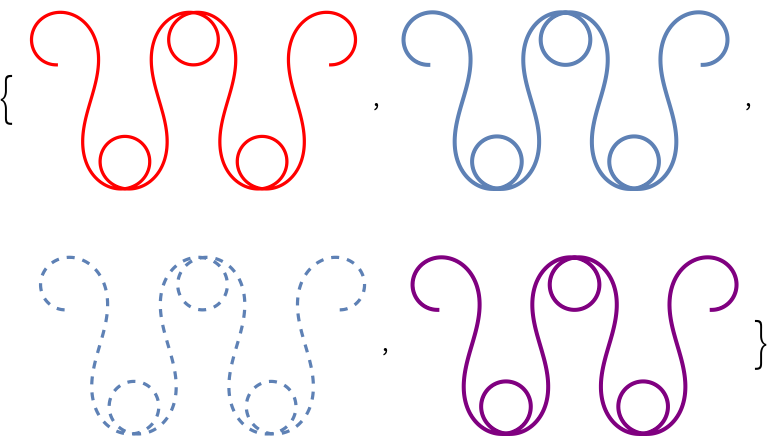By default, different styles are chosen for multiple curves:

 In:=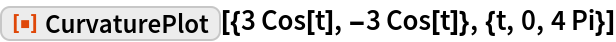Out=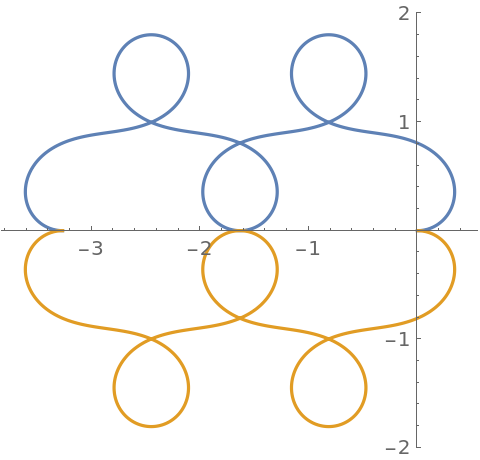Explicitly specify the style for different curves:

 In:=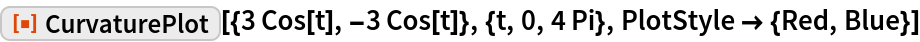Out=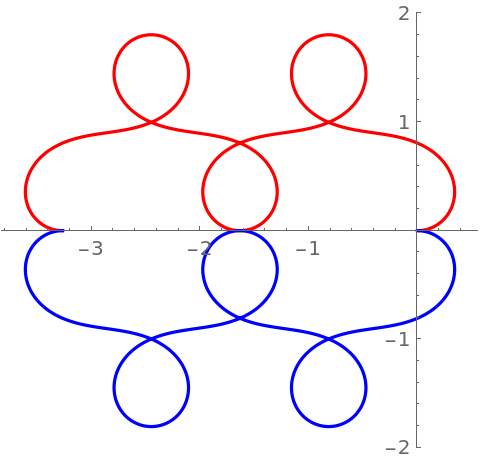#### PlotTheme

Use a marketing theme:

 In:=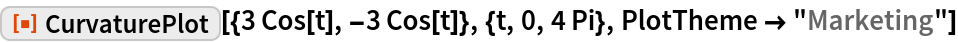Out=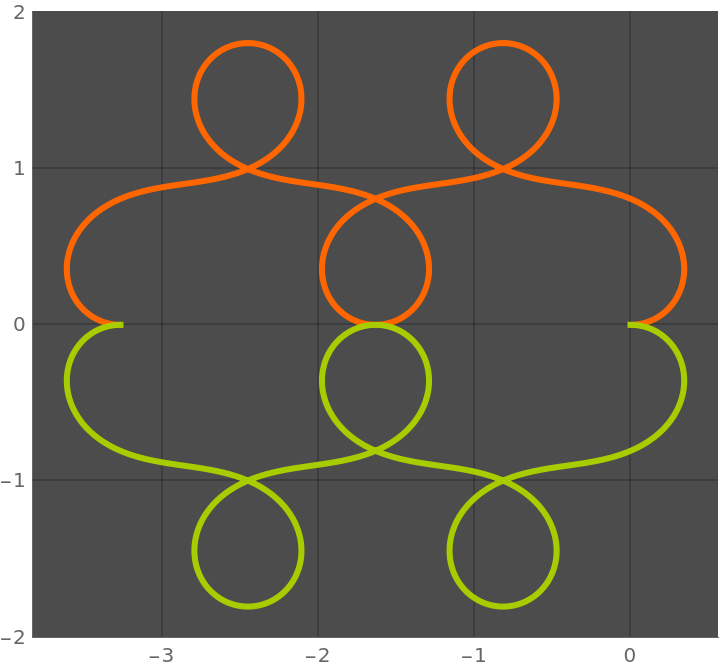#### WorkingPrecision

Evaluate functions using machine-precision arithmetic:

 In:=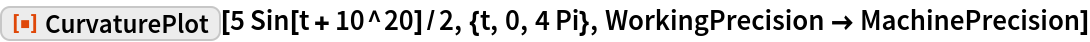Out=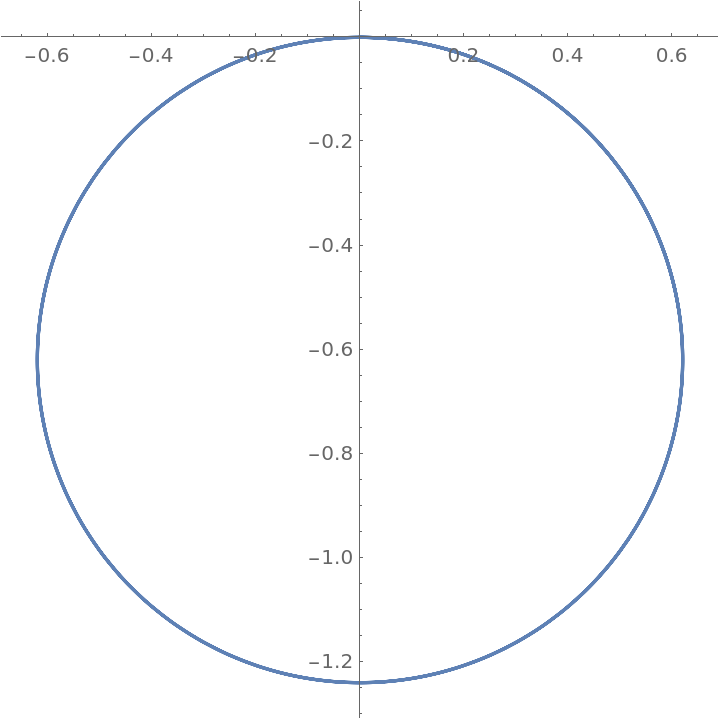Evaluate functions using arbitrary-precision arithmetic:

 In:=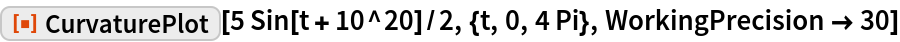Out=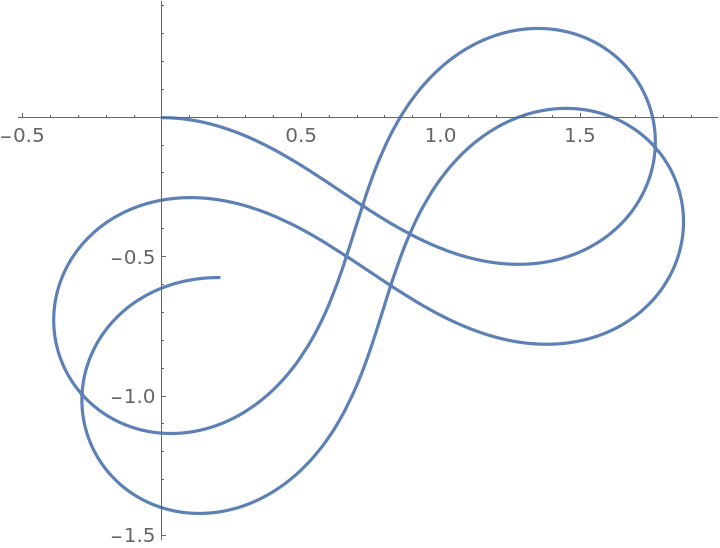### Possible Issues

More steps are needed in the integration:

 In:=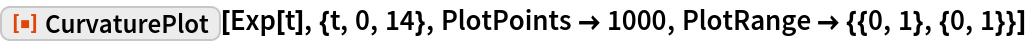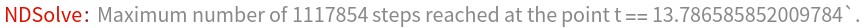Out=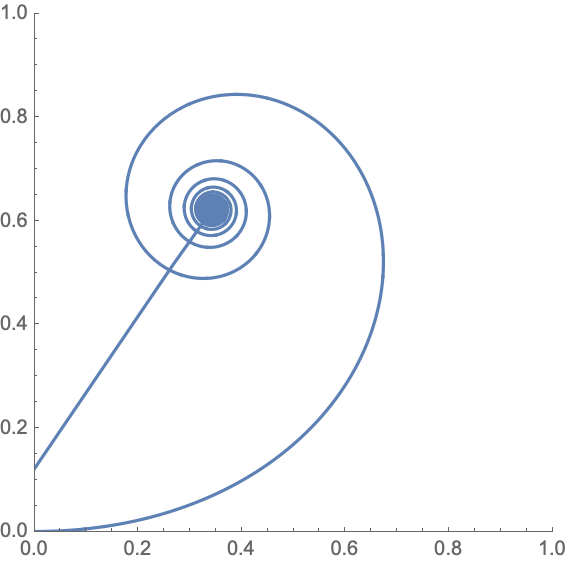Supply a larger MaxSteps option:

 In:=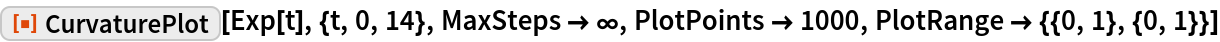Out=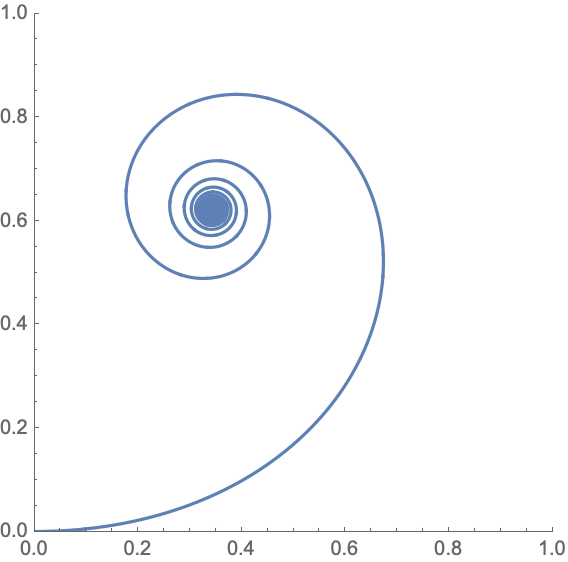### Neat Examples

Plot an increasingly curvy curve:

 In:=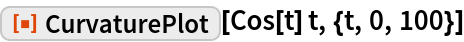Out=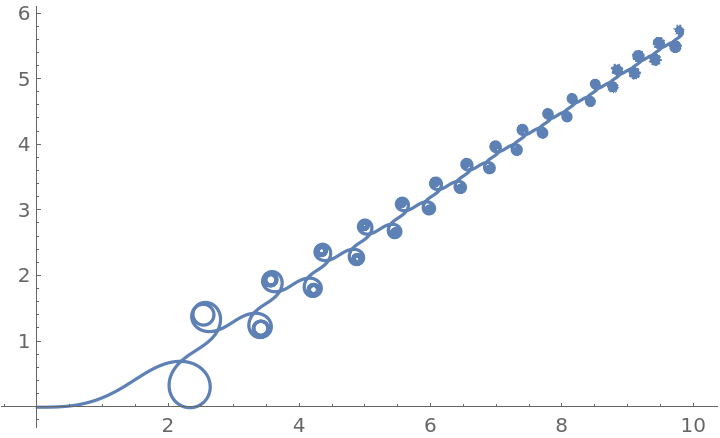Elementary function can lead to very complicated patterns:

 In:=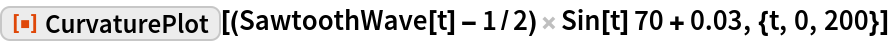Out=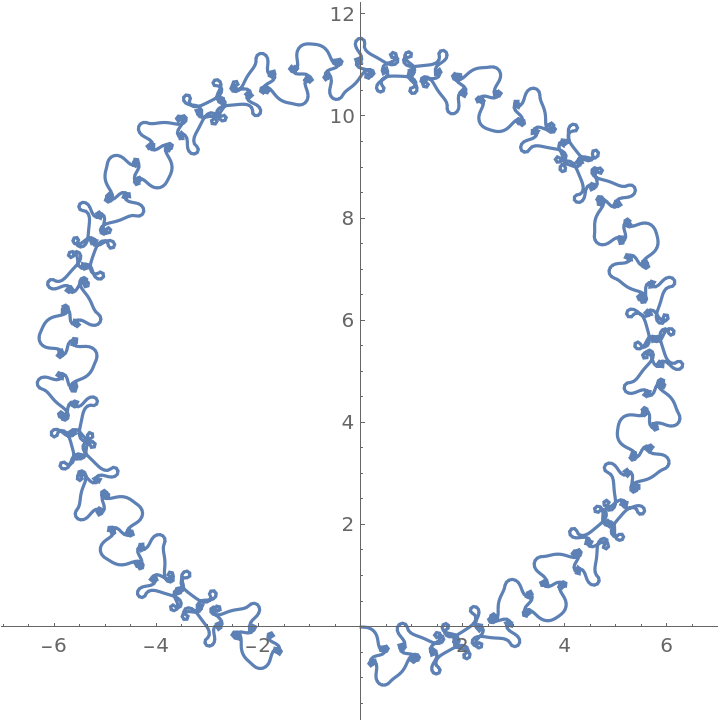Plot a bunch of connected circles:

 In:=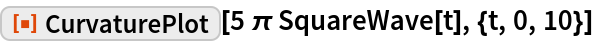Out=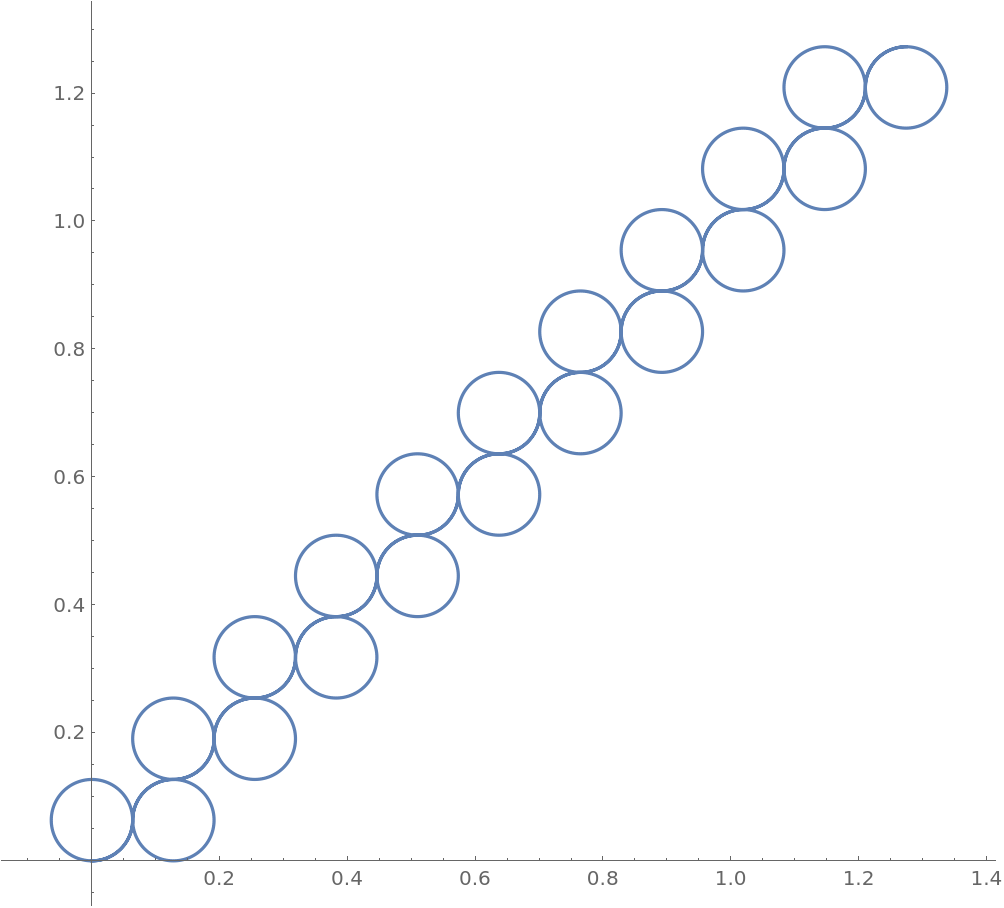Create intricate non-repeating patterns:

 In:=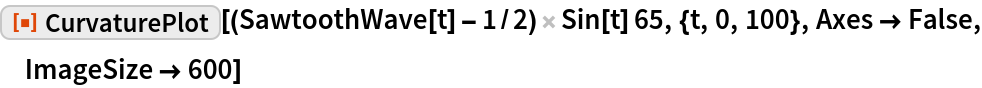Out=